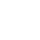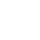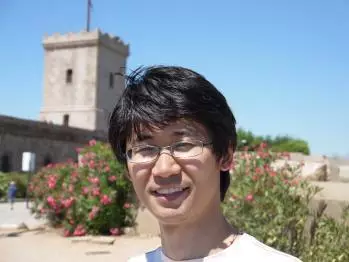# Structure Properties of Affine Sparsity Constraints2016年12月19日（星期一） 下午 2:00Structure Properties of Affine Sparsity ConstraintsHongbo Dong

Washington State University

Hongbo Dong has been an assistant professor at the Washington State University (USA) since 2013. He graduated with a PhD in Applied Mathematical and Computational Sciences from the University of Iowa in 2011, and worked as a research associate at the University of Wisconsin-Madison from 2011 to 2013. His research interests lie in the field of mathematical programming, and at the boundary between discrete and continuous optimization. Recently he is especially interested in non-convex optimization problems arising from the context of statistical learning. He has published research articles in both areas of optimization and statistics.

We consider a popular optimization formulation arising from variable selection in statistical modeling. Such optimization problems concern two criteria, one of which being a loss function that measures how well our model (to be estimated) fit the data available, while the other being a ``complexity” measure of the model. Well studied cases include the sparse regression, where many proposed convex (e.g., lasso) and non-convex methods have contributed greatly in advancing high-dimensional data analysis. However in many applications additional logic conditions are necessary, such as, certain variables must enter the model before some other variables, or variables selected should follow certain group structures, in order to develop models that are easily interpretable.
We propose a flexible constraint framework that can model (almost any kind of) logic conditions. It consists of a composition of linear systems of inequalities with binary indicator functions. Such constraints are not just nonconvex, but also involve discontinuity.
We address a few fundamental questions, such as closedness, set convergence (in Pompeiu-Hausdorff distance) of continuous approximations, and (algebraic) characterizations of tangent cones. Our results lay a mathematical foundation for algorithm design for this class of problems.
This is a (very) recent joint work with Jong-Shi Pang (USC) and Miju Ahn (USC).